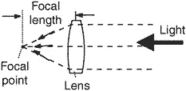# focal length

(redirected from Front focal length)
Also found in: Dictionary, Thesaurus, Medical.

## focal length

, distance
the distance from the focal point of a lens or mirror to the reflecting surface of the mirror or the centre point of the lens

## Focal length

A measure of the collecting or diverging power of a lens or an optical system. Focal length, usually designated f in formulas, is measured by the distance of the focal point (the point where the image of a parallel entering bundle of light rays is formed) from the lens, or more exactly by the distance from the principal point to the focal point. See Geometrical optics

The power of a lens system is equal to n/f, where n is the refractive index in the image space (n is usually equal to unity). A lens of zero power is said to be afocal. Telescopes are afocal lens systems. See Diopter, Lens (optics), Telescope

## focal length

Symbol: f . The distance from the center of a reflecting surface or refracting medium to the focal point or focus (see illustration). With a converging system, such as a paraboloid surface, a concave mirror or thin convex lens, the focus, F, is the point to which a narrow beam of light, radio waves, etc., from a distant object, i.e. a parallel beam closely aligned to the axis, is brought to a sharply defined or focused image. In a convex mirror or thin concave lens it is the point from which a parallel beam, made divergent by the mirror or lens, appears to diverge. If the two surfaces of a lens do not have identical curvatures the lens will have two different focal lengths and focal points, depending on which surface the light falls.

The focal plane is the plane through the focus, at right angles to the optical axis, in which the image of a distant object will be formed. In some cases, as in the Schmidt telescope, the image is focused on a curved surface – the focal surface – rather than a plane. See also effective focal length.

## focal length

[′fō·kəl ‚leŋkth]
(optics)
The distance from the focal point of a lens or curved mirror to the principal point; for a thin lens it is approximately the distance from the focal point to the lens. Also known as focal distance.

## focal lengthThe linear measurement along the optical axis between the center of the film and the center of the lens, with the camera focused at infinity.

## focal length

Focal length is the optical distance in millimeters between a camera lens and the sensor or film. It determines the height and width of the scene being captured, known as the "field of view."

A prime lens has one focal length and thus a fixed field of view. In order to change it, the photographer has to move closer or farther away from the subject. A zoom lens has a range from wide angle to telephoto.

Focal length is one of the two primary measurements of a camera lens. The other is the "aperture," which is the lens opening (see f-stop).

The Field of ViewThese photos were taken with an 18-70mm zoom lens on a digital SLR (see DSLR). At 18mm (top), the field of view is the greatest (wide angle). At 70mm (bottom), the scene is the narrowest (telephoto). This lens is equivalent to a 27-105mm lens on a 35mm SLR camera (see crop factor).

The Field of ViewThese photos were taken with an 18-70mm zoom lens on a digital SLR (see DSLR). At 18mm (top), the field of view is the greatest (wide angle). At 70mm (bottom), the scene is the narrowest (telephoto). This lens is equivalent to a 27-105mm lens on a 35mm SLR camera (see crop factor).
Site: Follow: Share:
Open / Close